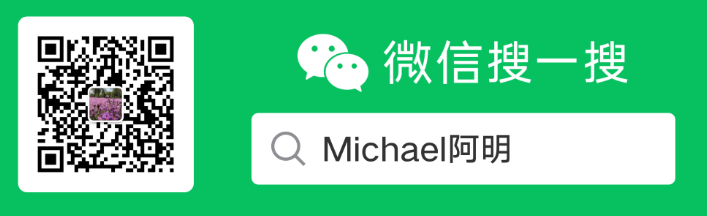# LeetCode 1087. 字母切换（回溯）

## 1. 题目

示例 1：

1 <= S.length <= 50



## 2. 解题

class Solution {
vector<string> ans;
public:
vector<string> expand(string S) {
vector<vector<char>> str;
vector<char> t;
int count = 0;
for(int i = 0; i < S.size(); ++i)
{
if(isalpha(S[i]))
t.push_back(S[i]);
else if(S[i] == '}' || (S[i] == '{' && !t.empty()))
{
if(S[i] == '}') count++;
sort(t.begin(), t.end());
str.push_back(t);
t.clear();
}
}
if(!t.empty())
{
sort(t.begin(), t.end());
str.push_back(t);
}
if(count == 0) return {S};//没有花括号，直接返回
dfs(str, "", 0);
return ans;
}

void dfs(vector<vector<char>>& str, string s, int i)
{
if(i == str.size())
{
ans.push_back(s);
return;
}
for(int j = 0; j < str[i].size(); ++j)
dfs(str, s+str[i][j], i+1);
}
};


8 ms 8.2 MB03-132567
09-2722906-16212
06-17155
09-1629
12-02171
03-273万+
06-1628
06-15498
11-131091